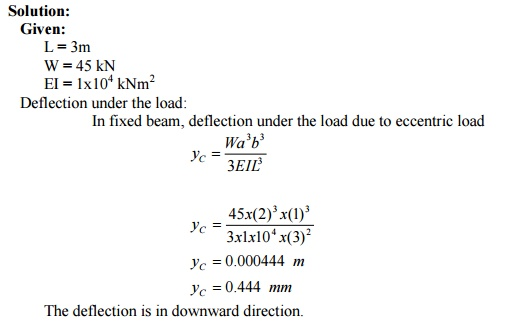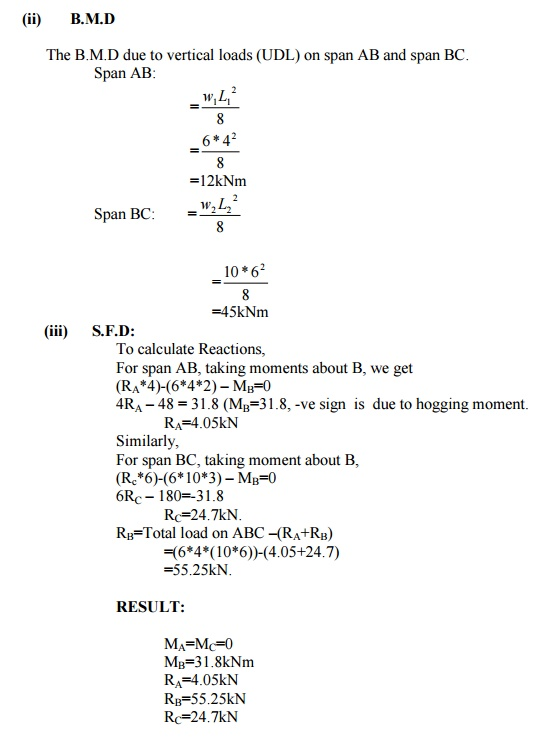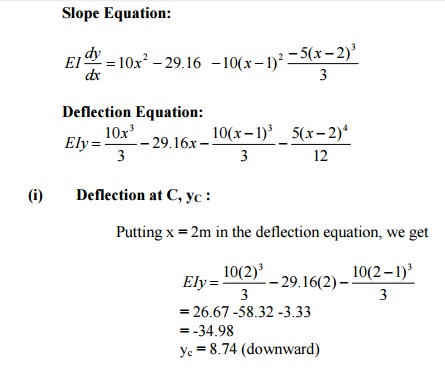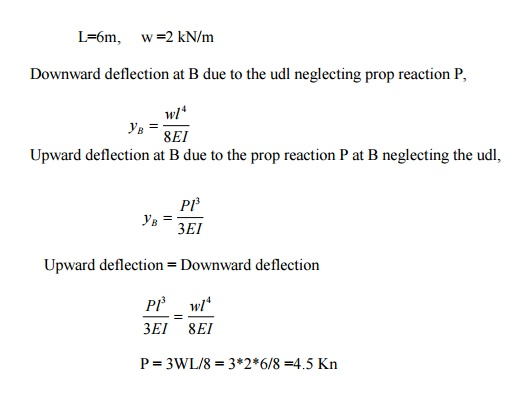Home | | Strength of Materials II | Solved Problems: Civil - Strength of Materials - Indeterminate Beams

# Solved Problems: Civil - Strength of Materials - Indeterminate Beams

Civil - Strength of Materials - Indeterminate Beams

A fixed beam AB of length 6m carries point load of 160 kN and 120 kN at a distance of 2m and 4m  from the left end A. Find the fixed end moments and the reactions at the supports. Draw B.M and  S.F diagrams.A fixed beam AB of length 6m carries two point loads of 30 kN each at a distance of 2m  from the both ends. Determine the fixed end moments and draw the B.M diagram.Find the fixing moments and support reactions of a fixed beam AB of length 6m, carrying a uniformly  distributed load of 4kN/m over the left half of the span.What are the fixed end moments for a fixed beam of length 'L  load 'w' at a distance 'a' from left end?

Fixed End Moment:A fixed beam of length 5m carries a uniformly distributed load of 9 kN/m run over the  entire span. If I = 4.5x10-4 m4 and E = 1x107 kN/m2, find the fixing moments at the ends and deflection at the centre.A fixed beam AB, 6m long is carrying a point load of 40 kN at its center. The M.O.I of the  beam is 78 x 106 mm4 and value of E for beam material is 2.1x105 N/mm2. Determine (i)  Fixed end moments at A and B.A fixed beam AB of length 3m is having M.O.I I = 3 x 106 mm4 and value of E for beam  material is 2x105 N/mm2. The support B sinks down by 3mm. Determine (i) fixed end  moments at A and B.A fixed beam AB, 3m long is carrying a point load of 45 kN at a distance of 2m from A. If  the flexural rigidity (i.e) EI of the beam is 1x104kNm2. Determine (i) Deflection under the Load.A fixed beam of 5m span carries a gradually varying load from zero at end A to 10 kN/m at  end B. Find the fixing moment and reaction at the fixed ends.A continuous beam ABC covers two consecutive span AB and BC of lengths 4m and 6m,  carrying uniformly distributed loads of 6kN/m and 10kN/m respectively. If the ends A and  C are simply supported, find the support moments at A,B and C. draw also B.M.D and S.F.D.A continuous beam ABCD of length 15m rests on four supports covering 3 equal spans and carries  a uniformly distributed load of 1.5 kN/m length .Calculate the moments and reactions at the  supports. Draw The S.F.D and B.M.D.A continuous beam ABCD, simply supported at A,B, C and D is loaded as shown in fig.

Find the moments over the beam and draw B.M.D and S.F.D.(i)                B.M.D due to vertical loads taking each span as simply supported:

(ii) B.M.D due to support moments:

Since the beam is simply supported MA =MD = 0

a)     For spans AB and BC

Using the theorem of three moments draw the shear force and bending moment diagrams  for the following continuous beam.A beam AB of 4m span is simply supported at the ends and is loaded as shown in fig.

Determine (i) Deflection at C (ii) Maximum deflection (iii) Slope at the end A.

E= 200 x 106 kN/m2 and I = 20 x 10-6 m49. A continuous beam is shown in fig. Draw the BMD indicating salient points.10. A cantilever beam AB of span 6m is fixed at A and propped at B. The beam carries a udl of  2kN/m over its whole length. Find the reaction at propped end.Study Material, Lecturing Notes, Assignment, Reference, Wiki description explanation, brief detail
Civil : Strength of Materials : Indeterminate Beams : Solved Problems: Civil - Strength of Materials - Indeterminate Beams |

Related Topics